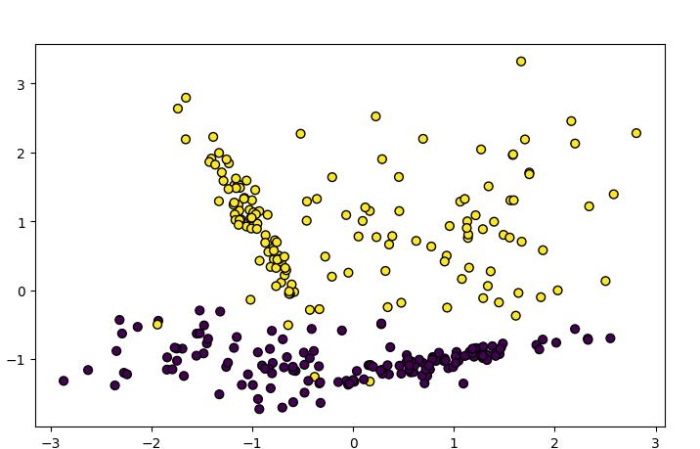# How to build Naive Bayes classifiers using Python Scikit-learn?

Naïve Bayes classification, based on the Bayes theorem of probability, is the process of predicting the category from unknown data sets. Scikit-learn has three Naïve Bayes models namely,

• Gaussian Naïve Bayes
• Bernoulli Naïve Bayes
• Multinomial Naïve Bayes

In this tutorial, we will learn Gaussian Naïve Bayes and Bernoulli Naïve Bayes classifiers using Python Scikit-learn (Sklearn).

## Gaussian Naïve Bayes Classifier

Gaussian naïve bayes classifier is based on a continuous distribution characterized by mean and variance.

With the help of an example, let’s see how we can use the Scikit-Learn Python ML library to build a Gaussian Naïve Bayes classifier.

For this example, we will be using Gaussian Naïve Bayes model which assumes that the data for each label is drawn from a simple Gaussian distribution. The dataset we will be using is the Breast Cancer Wisconsin Diagnostic Database.

### Example

# Importing the necessary packages
import sklearn

labelnames = DataSet['target_names']
labels = DataSet['target']
featurenames = DataSet['feature_names']
features = DataSet['data']

# Organizing dataset into training and testing set
# by using train_test_split() function
from sklearn.model_selection import train_test_split
train, test, train_labels, test_labels = train_test_split(features,labels,test_size = 0.30, random_state = 300)

# Model evaluation by using Naïve Bayes algorithm.
from sklearn.naive_bayes import GaussianNB

# Let's initializing the model:
NBclassifier = GaussianNB()

# Train the model:
NBmodel = NBclassifier.fit(train, train_labels)

# Making predictions by using pred() function:
NBpreds = NBclassifier.predict(test)
print("The predictions are:\n", NBpreds[:15])

# Finding accuracy of our Naive Bayes classifier:
from sklearn.metrics import accuracy_score
print("Accuracy of our classifier is:", accuracy_score(test_labels, NBpreds) *100)


### Output

It will produce the following output −

The predictions are:
[0 0 1 1 0 0 0 1 1 1 1 1 0 1 0]
Accuracy of our classifier is: 93.56725146198829


## Bernoulli Naive Bayes Classifier

Bernoulli Naïve Bayes classifier is a binary algorithm. It is useful when we need to check whether a feature is present or not.

With the help of an example, let’s see how we can use the Scikit-Learn Python ML library to build a Bernoulli Naïve Bayes classifier.

### Example

In the below giving example, we will be using scikit-learn python library to implement Bernoulli Naïve Bayes algorithm on a dummy dataset.

from sklearn.datasets import make_classification
# Importing libraries
from sklearn.datasets import make_classification
import matplotlib.pyplot as plt

# Creating the classification dataset with one informative feature and one cluster per class
nb_samples = 300
X, Y = make_classification(n_samples=nb_samples, n_features=2, n_informative=2, n_redundant=0)

# Plotting the dataset
plt.figure(figsize=(7.50, 3.50))
plt.subplot(111)
plt.scatter(X[:, 0], X[:, 1], marker="o", c=Y, s=40, edgecolor="k")
plt.show()


### Output

We will get the dummy dataset as follows −### Example

Now, let’s build Bernoulli Naïve bayes classifier on this dummy dataset −

# Importing libraries
from sklearn.datasets import make_classification
import numpy as np

# Model evaluation by using Bernoulli Naïve Bayes algorithm.

# Import Bernoulli Naive bayes from sklearn
from sklearn.naive_bayes import BernoulliNB

# Organizing dataset into training and testing set
# by using train_test_split() function
from sklearn.model_selection import train_test_split

# Creating the classification dataset with one informative feature and one cluster per class
nb_samples = 300
X, Y = make_classification(n_samples=nb_samples, n_features=2, n_informative=2, n_redundant=0)
X_train, X_test, Y_train, Y_test = train_test_split(X, Y, test_size=0.30)

# Let's initializing the model
B_NaiveBayes = BernoulliNB(binarize=0.0)

# Train the model
B_NaiveBayes.fit(X_train, Y_train)

# Making predictions by using pred() function
data = np.array([[0, 0], [0, 1], [1, 0], [1, 1]])
Preds=B_NaiveBayes.predict(data)
print(Preds)


### Output

It will produce the following output −

array([0, 0, 1, 1])